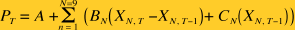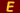# The Ecphorizer

 The Price Equation J. B. O'Donnell

#### Issue #46 (June 1985)

It is not a new thought that the value of money is related to the quantity of money. This tautological relationship has been known as long as economists have known the irrefutable law of supply and demand. That is, it is the relative availability of goods and services that determines their

The value of money can be controlled by monetary policy.

prices. Money is a good much like other goods. If there is more money available in [quoteright]the marketplace than the amount of goods and services available for sale then the value of money will fall. Likewise, if there is less money available its value will rise. Why, then, is it not a routine matter to reduce the quantity of money when inflation occurs and increase the quantity when deflation occurs?

The answers to this question are probably as numerous as the people who have attempted to solve this economic riddle. But I suspect they can all be summarized into one general problem. That problem is the lack of scientific evidence showing a direct relationship between prices and the quantity of money. That evidence is now available.

I believe there are two reasons why past efforts have not produced the required evidence. They are:

1. The failure to recognize the independence of various components of money supply; and
2. The failure to include money substitutes (credit) in the correl- ation equations used to demonstrate this relationship.

The method of demonstrating the relationship of money and prices is by use of the equation:where PT  = The current Consumer Price Index (or other measure
of money value);
Mx, T - DT  = A measure of the money supply (labeled M1, M2,
M3, etc.) for some time (T - DT) prior to the measure of CPI;
A and B = Correlation constants.

With this equation it has been demonstrated by many economists that there is apparently a one to three year time lag between a change in the money supply and its effect on prices. Because of this apparent time lag and the uncertainty of the quantity of goods and services that will be available in the future, it has been concluded that the quantity of money needed to control prices cannot be determined. If, however, it can be shown that there is no time lag between changes in money and credit supplies and their effects on prices then the value of money can be controlled by monetary policy in response to changes in a price index. And that is the discovery I call the price equation.

The price equation is based on monetary and credit data supplied by the Federal Reserve Bank of St Louis in March, 1985. The data covers the 145-month period from December, 1972, through December, 1984, and does not include the seasonal adjustments so often used to improve correlations.

The specific data items used for this version of the price equation are:

N = 1    X = Commercial and industrial loans
N = 2    X = Real-estate loans
N = 3    X = Individual loans
N = 4    X = Other bank loans (Total - (X1 + X2 + X3))
N = 5    X = Bank-held Government securities
N = 6    X = Other bank securities
N = 7    X = Demand deposits
N = 8    X = Other checkable deposits
N = 9    X = Ml - (X7 + X8)

These data items were correlated with the Consumer Price Index without the use of a time lag. The price equation used is:That is, the price equation computes the value of the Consumer Price Index using only the value of the data items for the immediately preceding month and the changes occurring during the month.

The correlation constants used are:

A = 93.720648

N = 1     B = -0.31135555      C = -0.18010571
N = 2     B = 0.39168323      C = 1.1803416
N = 3     B = -0.72960439      C = -0.69523094
N = 4     B = -0.011406676      C = 0.050865631
N = 5     B = -0.24121661      C = 0.016463938
N = 6     B = 0.14891817      C = 0.28337594
N = 7     B = -0.11748317      C = -0.34839097
N = 8     B = -0.046350728      C = -0.29043109
N = 9     B = 0.096110178      C = 0.72907324

The results of this correlation are:

Correlation coefficient (R) = 0.99928886
Standard deviation (S) = 2.4437912
Degrees of freedom (DF) = 125

With this correlation· it is obvious that there is essentially an immediate response of the price level (CPI) to changes in the quantity of money and money substitutes. It is, therefore, just as obvious that the value of money can be controlled by monetary policy. It is not as obvious that the goal of monetary policy should be stable prices. There is a substantial belief that there is a relationship between inflation and employment based on the Phillips Curve.

In my book Confessions of a Socialist-Libertarian I demonstrate the fallacy of the Phillips Curve and the real cause of unemployment. However, here I must limit my analysis to the price equation.

The method of stabilizing the value of money by monetary policy is as follows:

1. The Federal Reserve System should set bank reserve requirements as low as possible consistent with maintaining a constant value of the  CPI.
2. Bank reserve requirements should be increased any time the CPI is above the target value for two or more consecutive months.
3. A  measure of daily price changes should be maintained to guide Federal Reserve System Open Market Operations.
4. The Federal Reserve System should conduct its Open Market Operations so as to maintain a constant value of the CPI using the guidance provided by the pseudo-CPI.